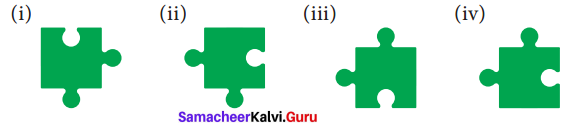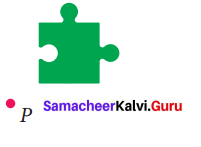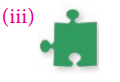# Samacheer Kalvi 7th Maths Solutions Term 3 Chapter 4 Geometry Ex 4.1

Students can Download Maths Chapter 4 Geometry Ex 4.1 Questions and Answers, Notes Pdf, Samacheer Kalvi 7th Maths Book Solutions Guide Pdf helps you to revise the complete Tamilnadu State Board New Syllabus and score more marks in your examinations.

## Tamilnadu Samacheer Kalvi 7th Maths Solutions Term 3 Chapter 4 Geometry Ex 4.1

Question 1.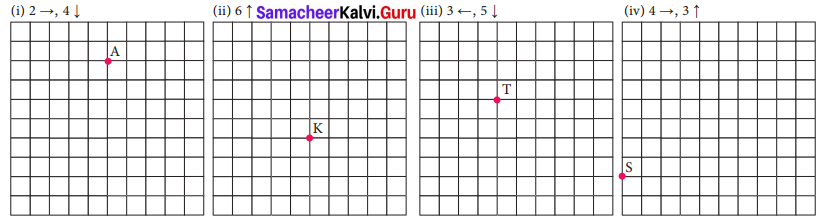Solution: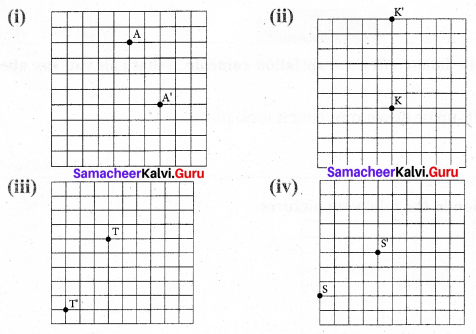Question 2.
How is the pre-image translated to the image?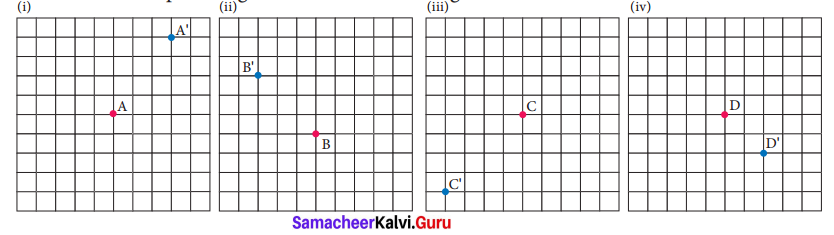Solution:
(i) 3 →, 4↑
(ii) 3 ←, 3↑
(iii) 4 ←, 4↓
(iv) 2 ←, 2↓

Question 3.
Find the image of the given triangle with given translation.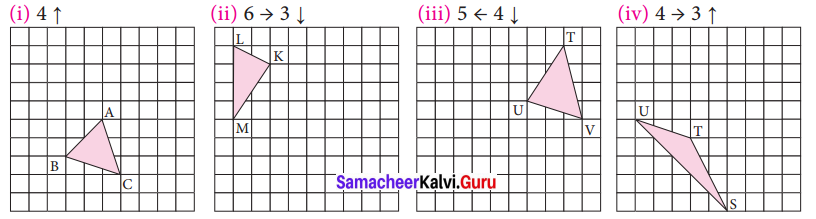Solution: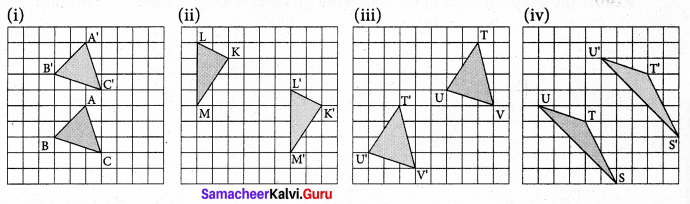Question 4.
Reflect the shape with given line of reflection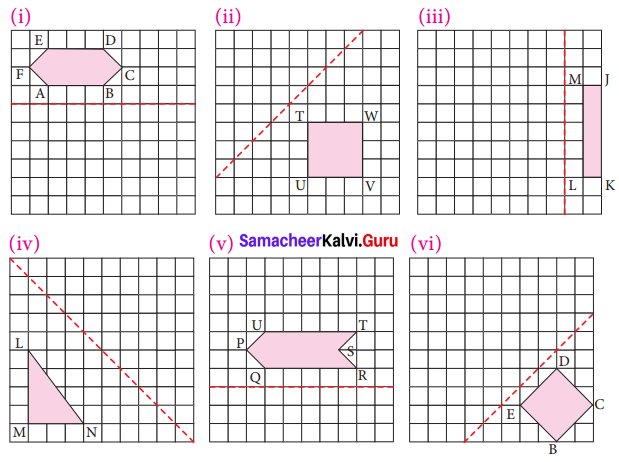Solution: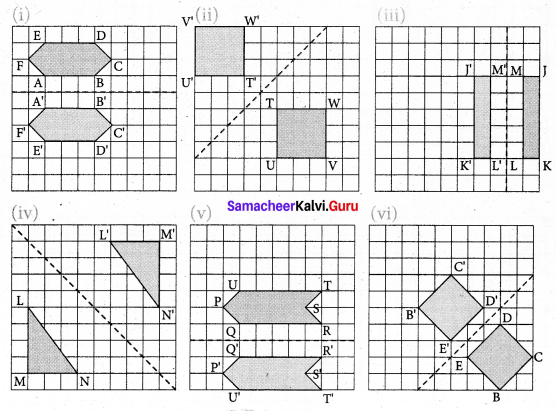Question 5.
Reflect the shape in each of the following pictures with given line of reflection.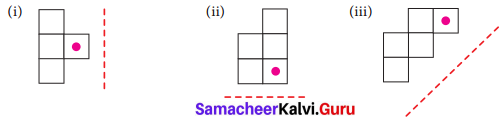Solution: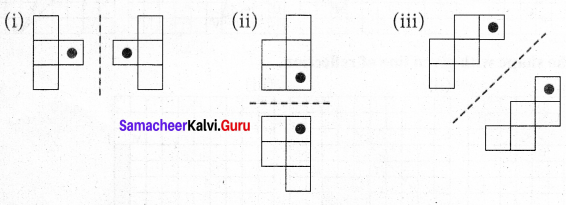Question 6.
Rotate the preimages in each case as directed about the red point.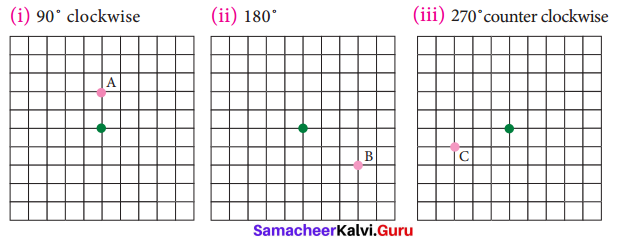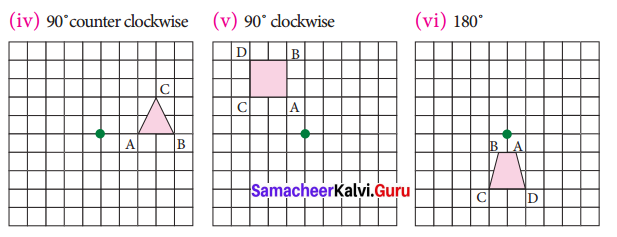Solution: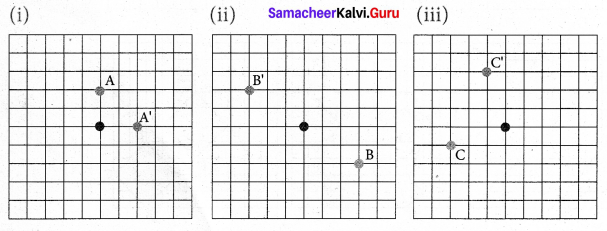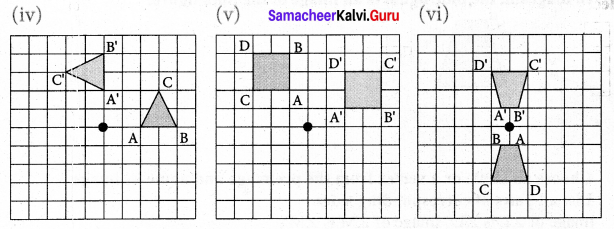Identify the transformation:

Question 7.Solution:
ReflectionQuestion 8.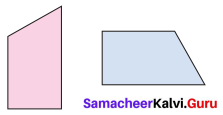Solution:
RotationQuestion 9.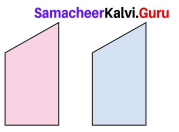Solution:
Translation

Question 10.
A pool of fish translates from point F to point D.
a. Describe the translation of the pool of fish.
b. Can the fishing boat make the same translation? Explain.
c. Describe a translation the fishing boat could make to get to point D.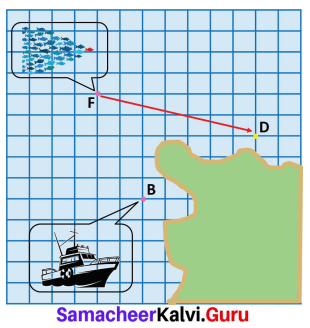Solution:
(a) Translation of pool of fish is 7 →, 2↓
(b) No, the fishing boat will be landed on the island if translated.
(c) To get point D, the translation will be 5 →, 3↓Question 11.
Name the transformation that will map footprint A onto the indicated footprint.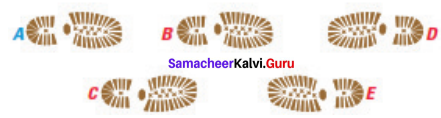(i) Footprint B
(ii) Footprint
(iii) Footprint D
(iv) Footprint E
Solution:
(i) It is translationQuestion 12.
In given diagram, the blue figure is an image of the pink figure.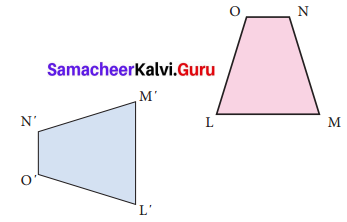(i) Choose an angle or a vertex from the preimage and name its image.
(ii) List all pairs of corresponding sides.
Solution:
(i) Image of ∠L is ∠L’, Image of ∠M is ∠M’,
Image of ∠N is ∠N’, Image of ∠O is ∠O’
Image of vertex L is L’, Image of vertex M is ∠M’
Image of vertex N is ∠N’, Image of vertex O is O’

(ii) Corresponding sides are LM and L’M’, MN and M’N’, NO and N’O’ and OL and O’L’

Question 13.
In the diagram at the right, the green figure is a translation image of the pink figure. Write a coordinate rule that describes the translation.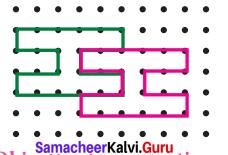Solution:
The rule bind here in 3→, 1↓

Objective Type Questions

Question 1.
A _______ is a turn about a point.
(i) Translation
(ii) Rotation
(iii) Reflection
(iv) Glide Reflection
(ii) Rotation

Question 2.
A _______ is a flip over a line.
(i) Translation
(ii) Rotation
(iii) Reflection
(iv) Glide Reflection
(iii) ReflectionQuestion 3.
A _______ is a slide; move without turning or flipping the shape.
(i) Translation
(ii) Rotation
(iii) Reflection
(iv) Glide Reflection
(i) Translation

Question 4.
The transformation used in the picture is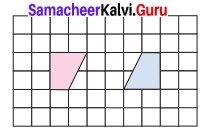(i) Translation
(ii) Rotation
(iii) Reflection
(iv) Glide Reflection
(ii) Rotation

Question 5.
The transformation used in the picture is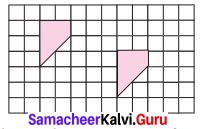(i) Translation
(ii) Rotation
(iii) Reflection
(iv) Glide Reflection